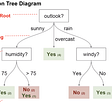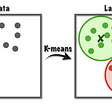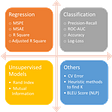# Evaluating Metrics for Multi-class Classification and Implementations

This blog is a continuation of this post.

Till now, you went through the Binary Classification metrics. from now, we are gonna learn the metrics for Multi-class classification and in the next article, you’re gonna learn Multi-label classification and its metrics.

Multi-class Classification is nothing but solving a classification problem where we have Multiple classes( No. of classes > 2) in the target column. For example, Classification of fruit images into Apples, oranges, and bananas.

I’m attaching an example dataset here. In this dataset, Development Index column is the target which has Multiple classes( No. of classes > 2).

As we learned the metrics in binary classification metrics, with them, we can make the multi-classification metrics.

There are 3 implementations of every binary classification metric in multi-classification metrics. you may understand when I write them…

For precision binary classification metric, in Multi-classification metrics, we have three versions,

1. Micro averaged precision
2. Macro averaged precision
3. Weighted precision

as precision, we have 3 versions of the recall binary classification metric in Multi-class classification.

1. Micro averaged recall
2. Macro averaged recall
3. Weighted recall

Like above, we can classify every binary classification metric as 3 versions and name them as multi-classification metrics.

For now, I’m gonna discuss precision and add the code of recall and all the remaining metrics gonna get built in the same way( same procedure followed for precision below). In the future post, I’m gonna show you how you can form ROC-AUC, in multi-class classification.

Note:- Don’t jump into anything, unless you know the basics. because it may eat your confidence and faith.

Let’s discuss the 3 versions of precision….

1. Micro averaged precision:- Here, first, we’re gonna find all the True positives for all of the classes and sum them to get total true positives from all classes. after that, we’re gonna find all the False positives for all of the classes and sum them to get total False positives from all classes. Now, calculate the precision for the total true positives and false positives.

2. Macro averaged precision:- Here, we are gonna find the precisions for different classes in the target column, and then average them to get the final precision.

3. Weighted precision:- Here, weighted precision is the same as Macro averaged precision, but the difference is Here, it depends and weighs based on the No of samples present in each class.

I know, I’ve given small definitions for each one, But that’s the definitions and code explains everything. So, check the definition and look at the required code for definition and know what’s happening in code, you’ll understand the things.

Keep in mind that, when the data is balanced, we can use the Macro version, but when data is imbalanced Micro and weighted versions are preferable.

Note that, the below code consists of all the 3 versions of precision.

`import pandasimport argparseimport numpyfrom collections import Counterfrom sklearn.linear_model import LogisticRegressionfrom sklearn.model_selection import train_test_splitfrom sklearn import metrics#there are 3 Types of precision in case of Multi-class classification. #1. Macro averaged precision#2. Micro averaged precision#3. Weighted precisiondef true_positive(y_true, y_pred):    tp = 0    for yt, yp in zip(y_true, y_pred):        if yt == 1 and yp == 1:            tp += 1    return tp    def true_negative(y_true, y_pred):    tn = 0    for yt, yp in zip(y_true, y_pred):        if yt == 0 and yp == 0:            tn += 1    return tn    def false_positive(y_true, y_pred):    fp = 0    for yt, yp in zip(y_true, y_pred):        if yt == 0 and yp == 1:            fp += 1    return fp    def false_negative(y_true, y_pred):    fn = 0    for yt, yp in zip(y_true, y_pred):        if yt == 1 and yp == 0:            fn += 1    return fndef precision(y_test, y_pred):    tp =true_positive(y_test, y_pred)    fp = false_positive(y_test, y_pred)    try:        return(tp/(tp+fp))    except ZeroDivisionError:        return 0def Macro_averaged_precision(y_test, predictions):    precisions = []    for i in range(1,5):        temp_ytest = [1 if x == i else 0 for x in y_test]        temp_ypred = [1 if x == i else 0 for x in predictions]        print(temp_ypred)        print(temp_ytest)        prec = precision(temp_ytest, temp_ypred)        precisions.append(prec)        return (sum(precisions)/len(precisions))         def Micro_averaged_precision(y_test, predictions):    tp = 0    fp = 0    for i in range(1,5):        temp_ytest = [1 if x == i else 0 for x in y_test]        temp_ypred = [1 if x == i else 0 for x in predictions]        tp += true_positive(temp_ytest, temp_ypred)        fp += false_positive(temp_ytest, temp_ypred)    precisions = tp / (tp + fp)    return precisionsdef weighted_precision(y_test, predictions):    num_classes = len(numpy.unique(y_test))    #coutns for every class    precision = 0    for i in range(1, num_classes):        temp_ytest = [1 if x == i else 0 for x in y_test]        temp_ypred = [1 if x == i else 0 for x in predictions]        tp = true_positive(temp_ytest, temp_ypred)        fp = false_positive(temp_ytest, temp_ypred)                try:            preai = tp / (tp+fp)        except ZeroDivisionError:            preai = 0        weighted = preai*sum(temp_ytest)        precision += weighted    precision = precision/len(y_test)    return precisionif __name__ == "__main__":        data = pandas.read_csv("C:\\Users\\iamvi\\OneDrive\\Desktop\\Metrics_in_Machine_Learning\\development-index\\Development Index.csv")        train = data.drop(['Development Index'], axis = 1).values    test = data["Development Index"].valuesmodel = LogisticRegression()X_train, X_test, y_train, y_test = train_test_split(train, test, stratify = test)model.fit(X_train, y_train)    predictions = model.predict(X_test)print("Macro precision is:", Macro_averaged_precision(y_test, predictions))    print("Micro precision is:", Micro_averaged_precision(y_test, predictions))    print("Weighted precision is:", weighted_precision(y_test, predictions))    print("sklearn Macro", metrics.precision_score(y_test, predictions, average = "macro"))    print("sklearn Micro", metrics.precision_score(y_test, predictions, average = "micro"))    print("sklearn weighted", metrics.precision_score(y_test, predictions, average = "weighted"))`

You may find it difficult to see the code because of inconsistent indentations due to the medium interface, click here to see the code in Github.

This is how all the multi-class classification metrics are produced from binary classification metrics.

As I said, I’m gonna give you the code for recall which resembles precision only, and all the remaining metrics too.

`import pandasimport argparseimport numpyfrom collections import Counterfrom sklearn.linear_model import LogisticRegressionfrom sklearn.model_selection import train_test_splitfrom sklearn import metrics#there are 3 Types of recall in case of Multi-class classification. #1. Macro averaged recall#2. Micro averaged recall#3. Weighted recalldef true_positive(y_true, y_pred):    tp = 0    for yt, yp in zip(y_true, y_pred):        if yt == 1 and yp == 1:            tp += 1    return tp    def true_negative(y_true, y_pred):    tn = 0    for yt, yp in zip(y_true, y_pred):        if yt == 0 and yp == 0:            tn += 1    return tn    def false_positive(y_true, y_pred):    fp = 0    for yt, yp in zip(y_true, y_pred):        if yt == 0 and yp == 1:            fp += 1    return fp    def false_negative(y_true, y_pred):    fn = 0    for yt, yp in zip(y_true, y_pred):        if yt == 1 and yp == 0:            fn += 1    return fndef recall(y_test, y_pred):    tp = true_positive(y_test, y_pred)    fn = false_negative(y_test, y_pred)    return(tp/(tp+fn))def Macro_averaged_recall(y_test, predictions):    recalls = []    for i in range(1,5):        temp_ytest = [1 if x == i else 0 for x in y_test]        temp_ypred = [1 if x == i else 0 for x in predictions]        print(temp_ypred)        print(temp_ytest)        rec = recall(temp_ytest, temp_ypred)        recalls.append(rec)        return (sum(recalls)/len(recalls))         def Micro_averaged_recall(y_test, predictions):    tp = 0    tn = 0    for i in range(1,5):        temp_ytest = [1 if x == i else 0 for x in y_test]        temp_ypred = [1 if x == i else 0 for x in predictions]        tp += true_positive(temp_ytest, temp_ypred)        tn += true_negative(temp_ytest, temp_ypred)    recall = tp / (tp + tn)    return recalldef weighted_recall(y_test, predictions):    num_classes = len(numpy.unique(y_test))    #counts for every class    recall = 0    for i in range(1, num_classes):        temp_ytest = [1 if x == i else 0 for x in y_test]        temp_ypred = [1 if x == i else 0 for x in predictions]tp = true_positive(temp_ytest, temp_ypred)        tn = true_negative(temp_ytest, temp_ypred)                try:            rec = tp / (tp+tn)        except ZeroDivisionError:            rec = 0weighted = rec*sum(temp_ytest)recall += weightedrecall = recall/len(y_test)    return recallif __name__ == "__main__":        data = pandas.read_csv("C:\\Users\\iamvi\\OneDrive\\Desktop\\Metrics_in_Machine_Learning\\development-index\\Development Index.csv")        train = data.drop(['Development Index'], axis = 1).values    test = data["Development Index"].valuesmodel = LogisticRegression()X_train, X_test, y_train, y_test = train_test_split(train, test, stratify = test)model.fit(X_train, y_train)    predictions = model.predict(X_test)print("Macro recall is:", Macro_averaged_recall(y_test, predictions))    print("Micro recall is:", Micro_averaged_recall(y_test, predictions))    print("Weighted recall is:", weighted_recall(y_test, predictions))    print("sklearn Macro", metrics.recall_score(y_test, predictions, average = "macro"))    print("sklearn Micro", metrics.recall_score(y_test, predictions, average = "micro"))    print("sklearn weighted", metrics.recall_score(y_test, predictions, average = "weighted"))`

The definitions of 3 versions of precision will be applied to recall too.

After you implemented all the multi-class classification metrics as I said above in 3 versions, you can confidently say “ I know Multi-class classification”.😉

Check the Classification-Metrics Repository to get all the codes of concepts I explained. As I’m writing articles here, GitHub is gonna get updated with codes. So, keep a fork on that.

If something you want to add here or want me to explain a missing concept here, lemme know from here.

Happy Learning✌.

--

--

--

## More from Geek Culture

A new tech publication by Start it up (https://medium.com/swlh).

## Decision Tree.## GPT-3: Careful First Impressions## Kubeflow 0.3 Simplifies Setup & Improves ML Development## Deep Learning: Style Transfer## ML-K-means Clustering## Using Computer Vision and Clustering to Develop the Next Alpha Go## Classification Model Evaluation## It’s not easy being clean## Vishnu vardhan Varapalli

Software Engineer, working on real-time problems to minimize the errors.

## Deep Learning Techniques for Text Representation — Part 1## Predicting Medical Specialities from Transcripts: A Complete Walkthrough using ULMFiT## Word2Vec SkipGram with Math and Implementation## How to handle Imbalanced Data for Classification. Case study: Credit Card Fraud Detection